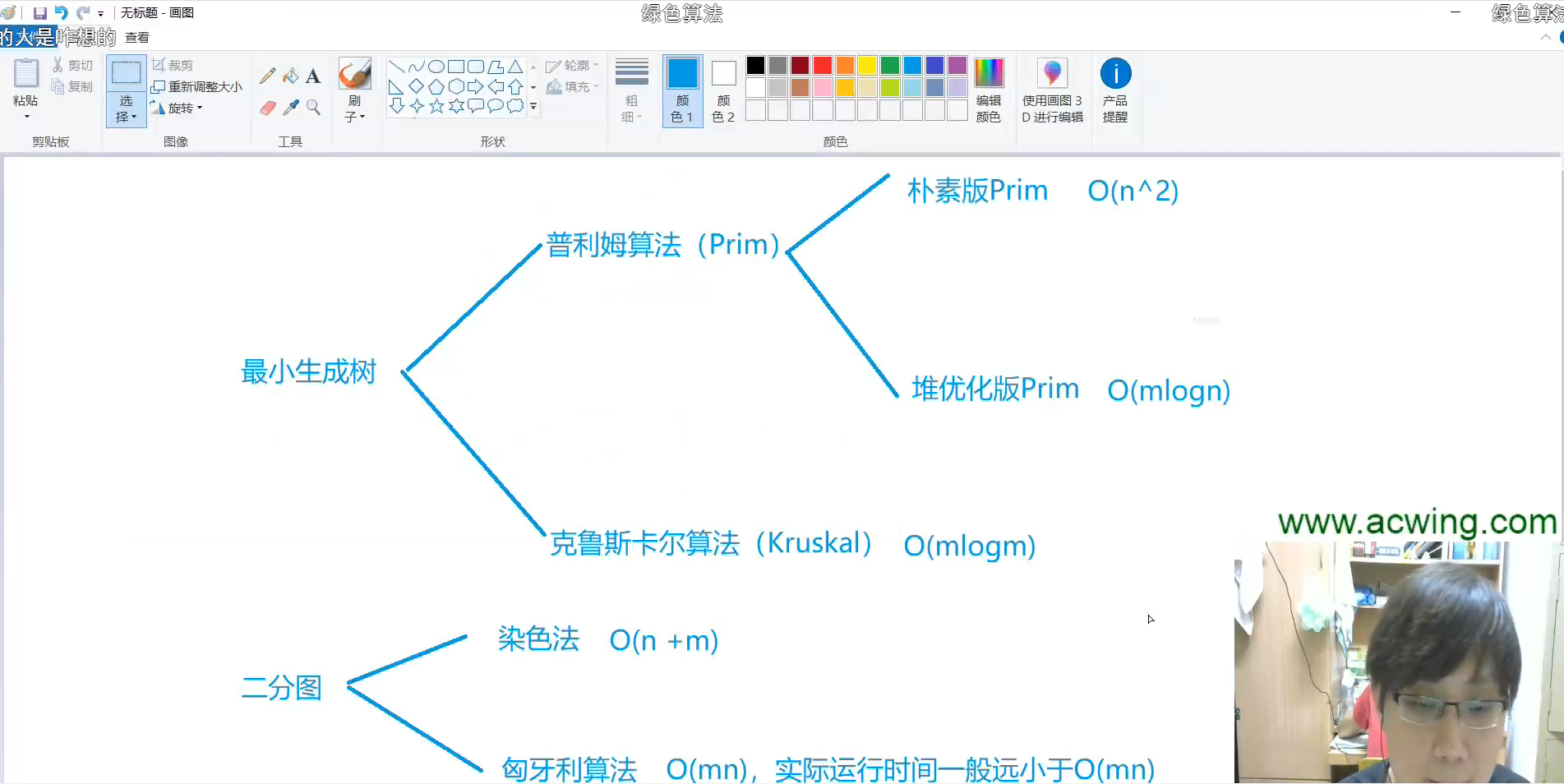# 二分图

+关注继续查看

### 什么是二分图

(X,Y)，并且图中的每条边（ij

### 关联的两个顶点i和j分别属于这两个不同的顶点集X和 Y ， 则称图G为一个二分图。1 匈牙利算法中，初始匹配我们可以设为空，然后用DFS或者BFS找增广路。找一条增广路的复杂度为OE），最多找V条增广路，故算法时间复杂度为OVE）。

2 只要有找到增广路，就让ans++，为什么呢，因为一条增广路总是有未匹配的边比匹配边多1，那么如果取反后就相当与匹配边多1，那么就是ans++。

/*DFS实现二分图的最大匹配*/
#include<iostream>
#include<cstdio>
#include<cstring>
#include<algorithm>
using namespace std;

#define MAXN 1010
int Nx , Ny;/*Nx Ny分别表示的是左右两边的最大的集合的个数*/
int G[MAXN][MAXN];
int Mx[MAXN] , My[MAXN];/*Mx , My分别表示的是左右两边是否和另一边相连*/
bool mark[MAXN];/*标记右边的点是否被匹配过*/

/*查找增广路*/
bool FindPath(int u){
/*枚举所有的右边集合的点*/
for(int i = 1 ; i <= Ny ; i++){/*注意这个地方下标是从0开始还是1*/
if(G[u][i] && !mark[i]){
mark[i] = true;
if(My[i] == -1 || FindPath(My[i])){
/*互换*/
My[i] = u;
Mx[u] = i;
return true;
}
}
}
return false;
}

/*求最大的匹配*/
int MaxMatch(){
int cnt = 0;
memset(Mx , -1 , sizeof(Mx));/*左边集合初始话为空*/
memset(My , -1 , sizeof(My));/*右边集合初始化为空*/
/*每一次都找左边一个未匹配的点进行找增广路*/
for(int i = 1 ; i <= Nx ; i++){/*注意这个地方下标是从0开始还是1*/
if(Mx[i] == -1){
memset(mark , 0 , sizeof(mark));
if(FindPath(i))
cnt++;
}
}
return cnt;
}
int main(){
/*建模*/
printf("%d\n" , MaxMatch());
return 0;
}

2 BFS实现二分图的最大匹配
#include<iostream>
#include<cstdio>
#include<cstring>
#include<algorithm>
using namespace std;

#define MAXN 1010

int Nx , Ny;/*左边集合和右边集合*/
int G[MAXN][MAXN];/*存储关系的矩阵*/
int Mx[MAXN] , My[MAXN];/*左边和右边点和另外边相连的编号*/
int pre[MAXN] , Q[MAXN];
int mark[MAXN];

int MaxMatch(){
int ans = 0;
int front , rear;
/*把左边和右边集合置为空*/
memset(Mx , -1 , sizeof(Mx));
memset(My , -1 , sizeof(My));
memset(mark , -1 , sizeof(mark));

for(int i = 1 ; i <= Nx ; i++){/*注意下标是从1开始还是0开始*/
if(Mx[i] == -1){
front = rear = 0;
Q[rear++] = i;
pre[i] = -1;

bool flag = 0;

while(front < rear && !flag){
int u = Q[front];
for(int v = 1 ; v <= Ny && !flag ; v++){/*注意下标是从1开始还是0开始*/
if(G[u][v] && mark[v] != i){
mark[v] = i;
Q[rear++] = My[v];
if(My[v] >= 0)
pre[My[v]] = u;
else{
flag = 1;
int d = u , e = v;
while(d != -1){
int t = Mx[d];
Mx[d] = e;
My[e] = d;
d = pre[d];
e = t;
}
}
}
}
front++;
}
if(Mx[i] != -1)
ans++;
}
}
return ans;
}

int main(){
/*建模*/
printf("%d\n" , MaxMatch());
return 0;
}|
2月前
|

【喜闻乐见，包教包会】二分图最大匹配:匈牙利算法（洛谷P3386）
【喜闻乐见，包教包会】二分图最大匹配:匈牙利算法（洛谷P3386）
22 0
|

28 0
|

54 0|

1204 0
|

1204 0